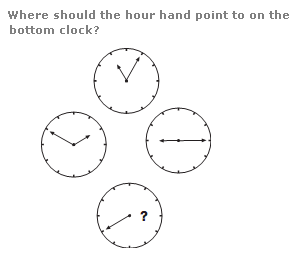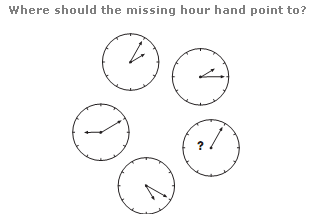# Puzzles - Clock puzzles

### Exercise :: Clock puzzlesAnswer : The hour hand points to the 4. Explanation : The sum of the values pointed to by the hands on each clock equals 12.

View Answer & ExplanationAnswer : To the 6 Explanation : Starting with the top left clockface and working clockwise around the others, the sum of the numbers pointed to by the 2 hands starts at 3 and increases by 2 each time.

View Answer & Explanation

#### Current Affairs 2019

Interview Questions and Answers